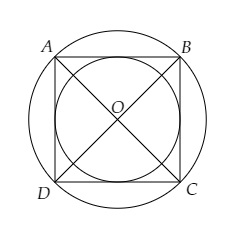# The side of a square is 10 cm. Find the area of circumscribed and inscribed circles.

Given:

The side of a square is 10 cm.

To do:

We have to find the area of circumscribed and inscribed circles.

Solution:

Let $ABCD$ be a square whose each side is $10\ cm$, $r_1$ be the radius of the circumscribed circle and $r_2$ be the radius of the inscribed circle.This implies,

$AB = BC = CD = DA = 10\ cm$

$AC$ and $BD$ are the diagonals of the square.

$\mathrm{AC}=\mathrm{BD}=\sqrt{2}\times10$

$=1.414 \times 10$

$=14.14 \mathrm{~cm}$

Radius of the circumscribed circle $\mathrm{r_1}=\frac{\mathrm{AC}}{2}$

$=\frac{14.1}{2}$

$=7.05 \mathrm{~cm}$

Radius of the inscribed circle $r_2=\frac{\mathrm{AB}}{2}$

$=\frac{10}{2}$

$=5 \mathrm{~cm}$

Area of the circumscribed circle $=\pi \mathrm{r_1}^{2}$

$=\frac{22}{7} \times(7.07)^{2}$

$=\frac{22}{7} \times 7.07 \times 7.07$

$=22 \times 1.01 \times 7.07$

$=157.0954$

$=157.1 \mathrm{~cm}^{2}$

Area of the inscribed circle $=\pi r_2^{2}$

$=\frac{22}{7} \times 5 \times 5 \mathrm{~cm}^{2}$

$=\frac{550}{7}$

$=78.57 \mathrm{~cm}^{2}$

The area of the circumscribed and the inscribed circles is $157.1\ cm^2$ and $78.57\ cm^2$ respectively.

Updated on: 10-Oct-2022

159 Views